# THE AUDITORY MODELING TOOLBOX

Applies to version: 1.1.0

Go to function

# DATA_LINDEMANN1986 - Data points from the Lindemann (1986a) paper

## Usage

data = data_lindemann1986_data(flag)


## Output parameters

 data the data points from the given figure as a matrix with x and y data or (in cases where no clear x values were given such as T/2) as a vector containing only the y data

## Description

data_lindemann1986(flag) returns data points from the Lindemann 1986 paper. The flag may be one of:

 'no_plot' Don't plot, only return data. This is the default. 'plot' Plot the data. 'fig11_yost' Return data from Fig. 11. with condition "yost". The data is ILD vs. lateral displacement (10 is max Displacement). 'fig11_sayers' Return data from Fig. 11. with condition "sayers". The data is ILD vs. lateral displacement (10 is max displacement). 'fig12_400' Return data from Fig. 12. for the 400 Hz pure tone. The data is ILD vs. ITD. 'fig12_600' Return data from Fig. 12. for the 600 Hz pure tone. The data is ILD vs. ITD. 'fig13' Return data from Fig. 13. The output data format is x-axis, -3dB, 3dB, 9dB, 15dB, 25dB. The data is ILD vs. ITD. 'fig16' Return data from Fig. 16. The data is ILD vs. ITD. 'fig17' Return data from Fig. 17. The output data format is x-axis, 0ms, 0.09ms, 0.18ms, 0.27ms. The data is ITD vs. ILD.

If no flag is given, the function will print the list of valid flags.

## Examples:

Figure 11 with the "yost" condition can be displayed using

data_lindemann1986('fig11_yost','plot');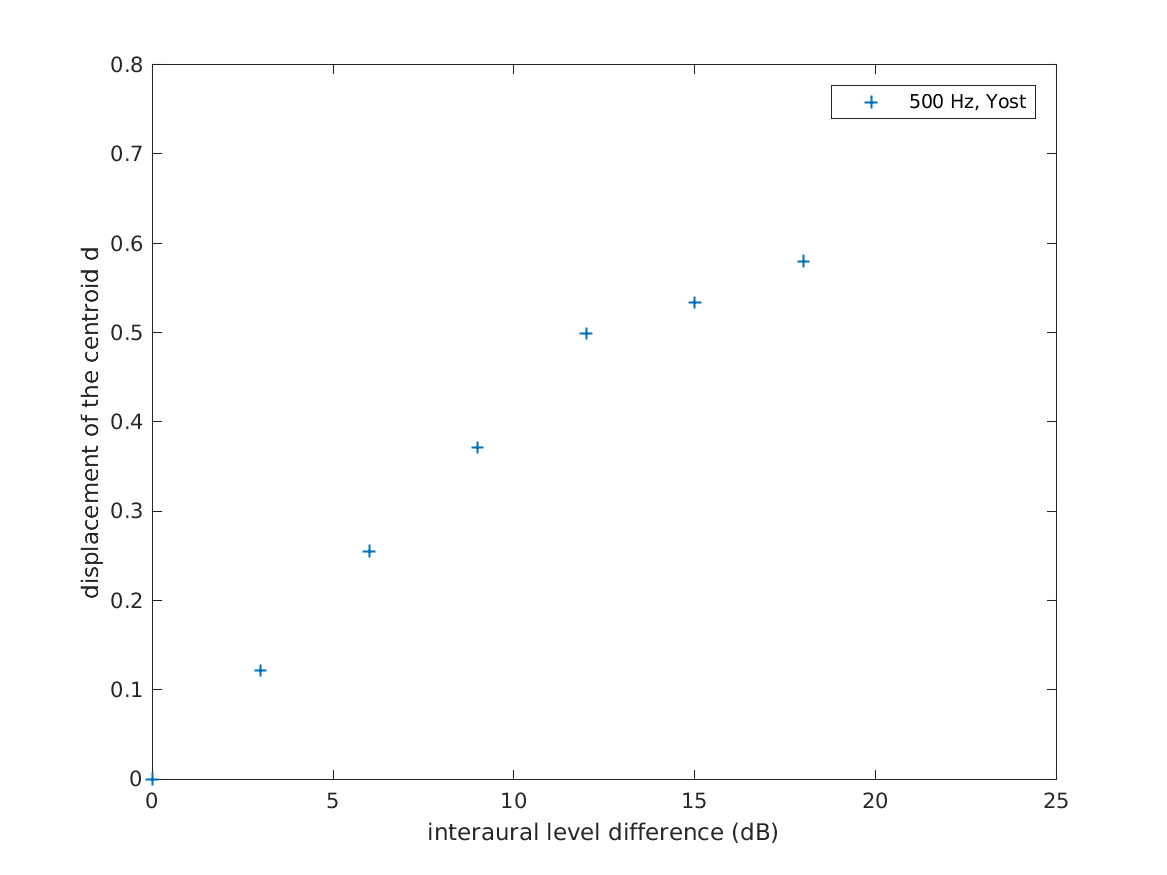Figure 11 with the "sayers" condition can be displayed using

data_lindemann1986('fig11_sayers','plot');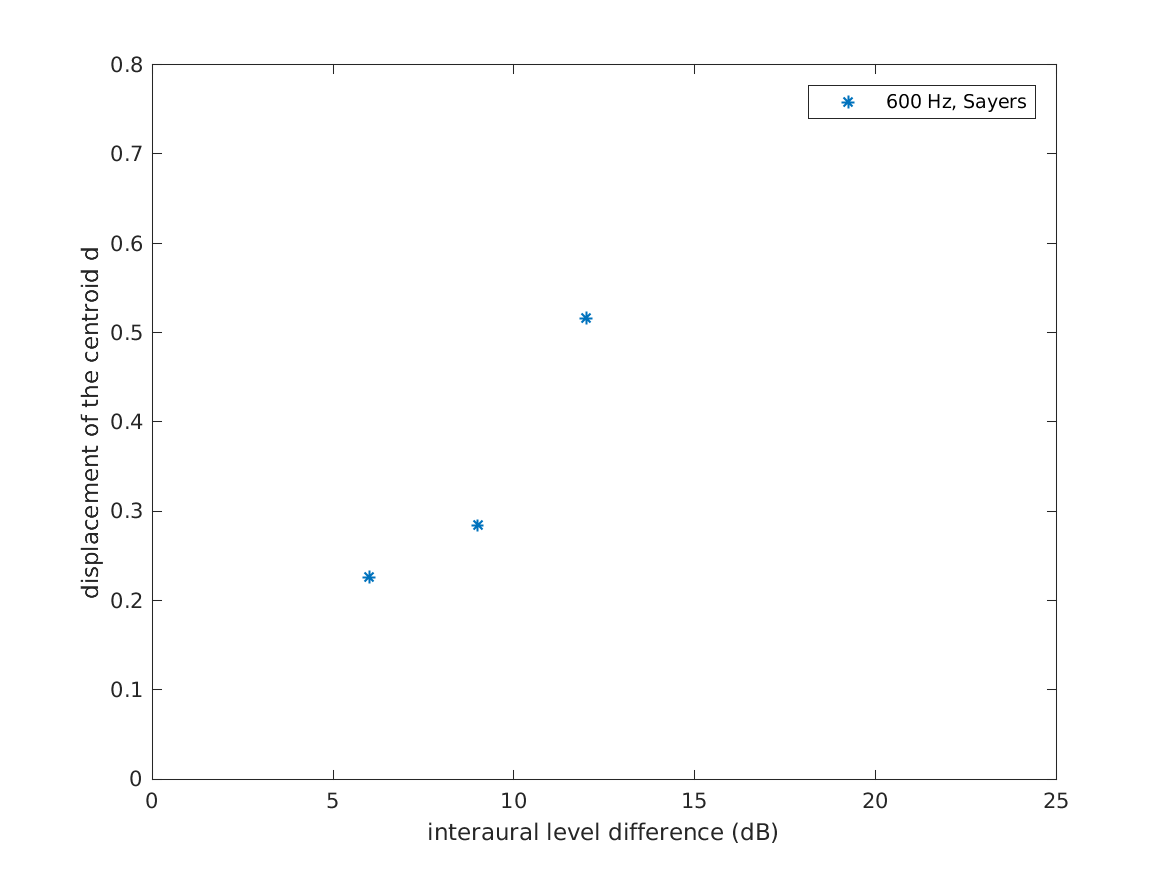Figure 12 for a 400 Hz pure tone can be displayed using

data_lindemann1986('fig12_400','plot');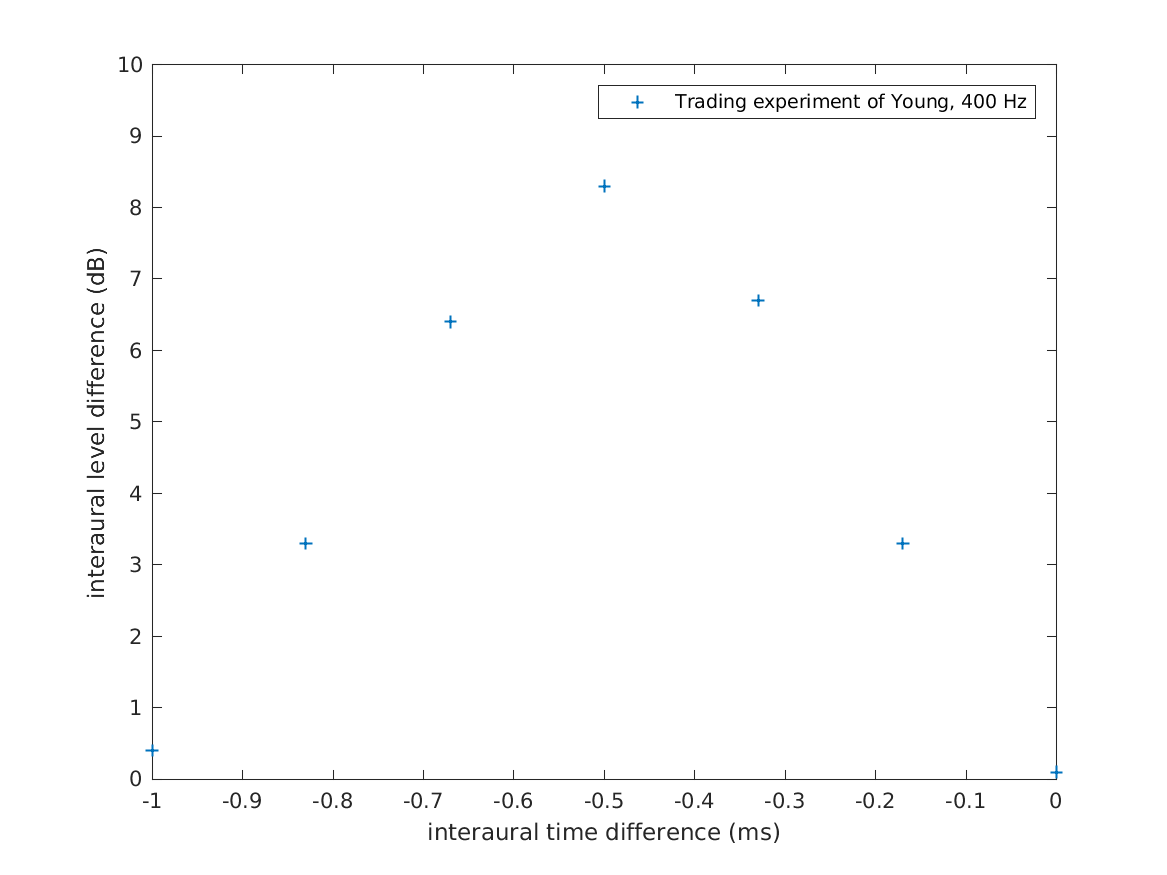Figure 12 for a 600 Hz pure tone can be displayed using

data_lindemann1986('fig12_600','plot');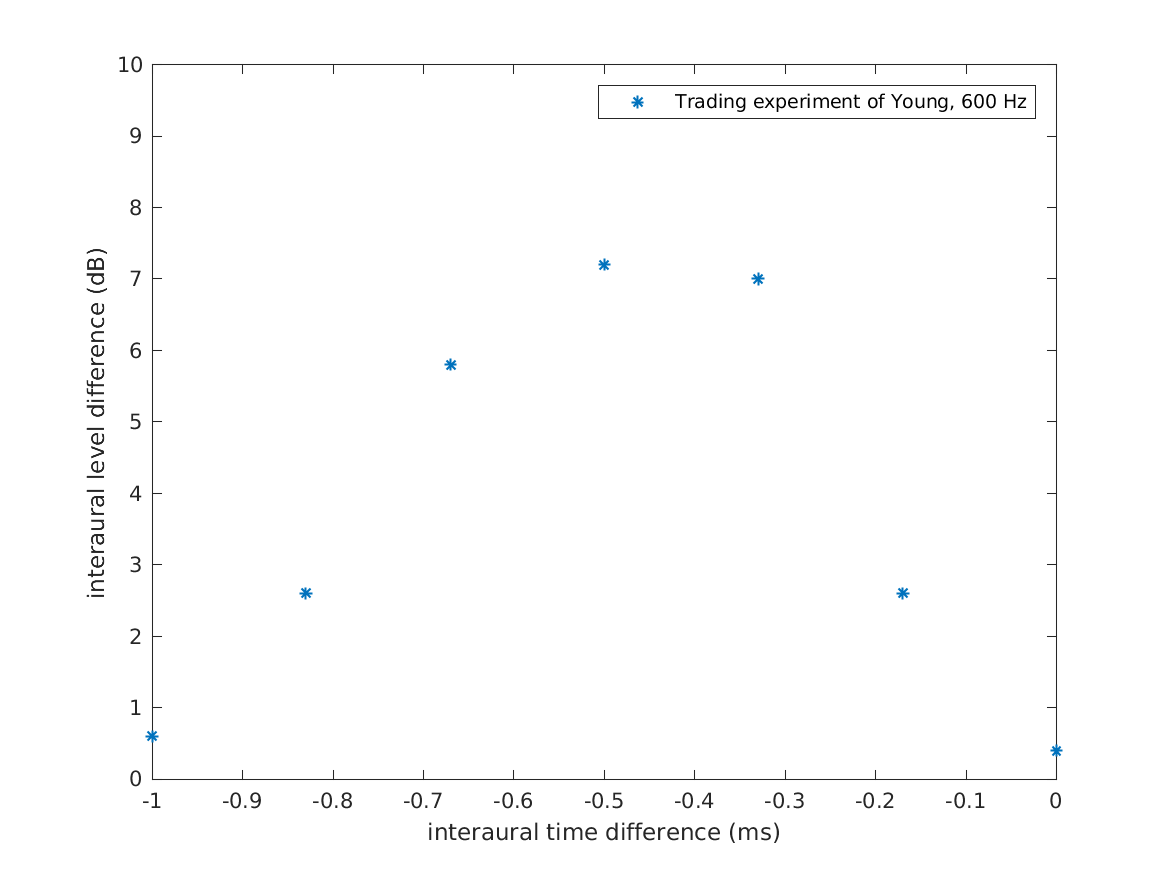Figure 13 can be displayed by using

data_lindemann1986('fig13','plot');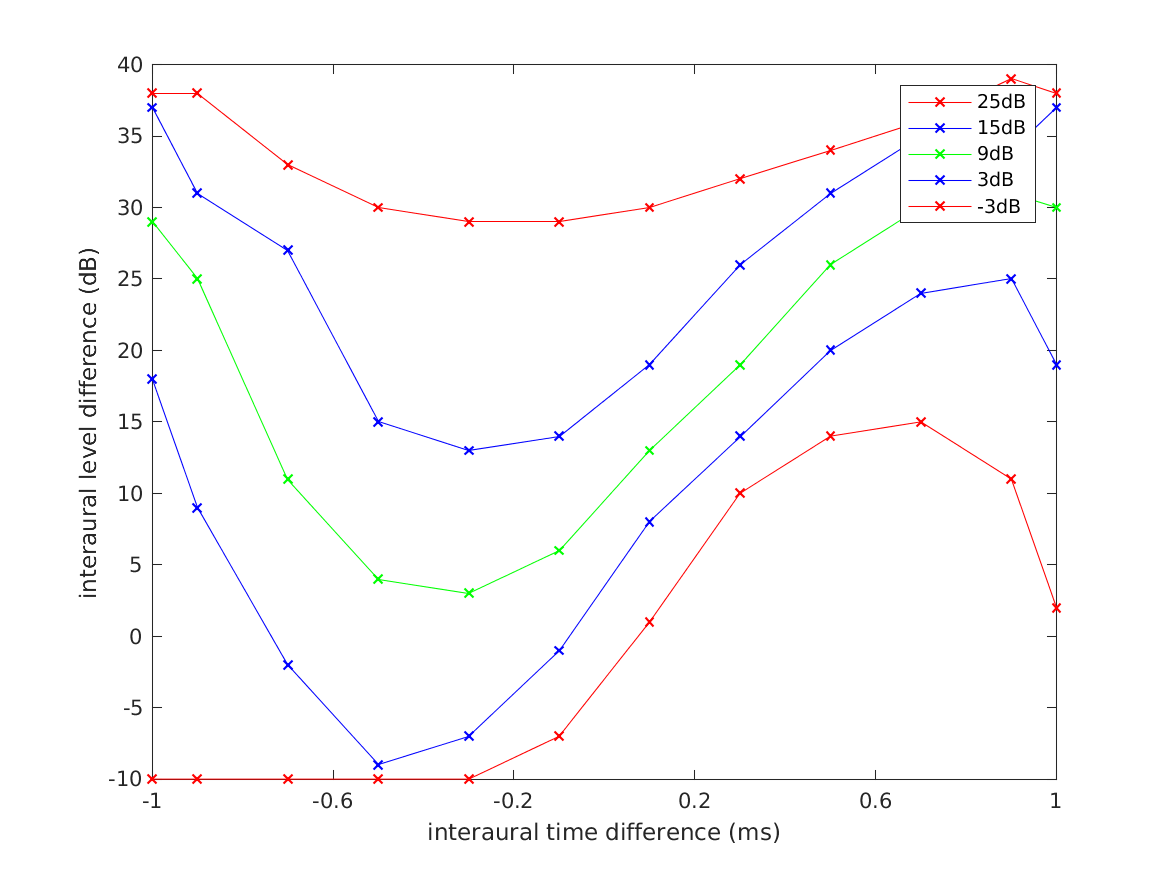Figure 16 can be displayed using

data_lindemann1986('fig16','plot');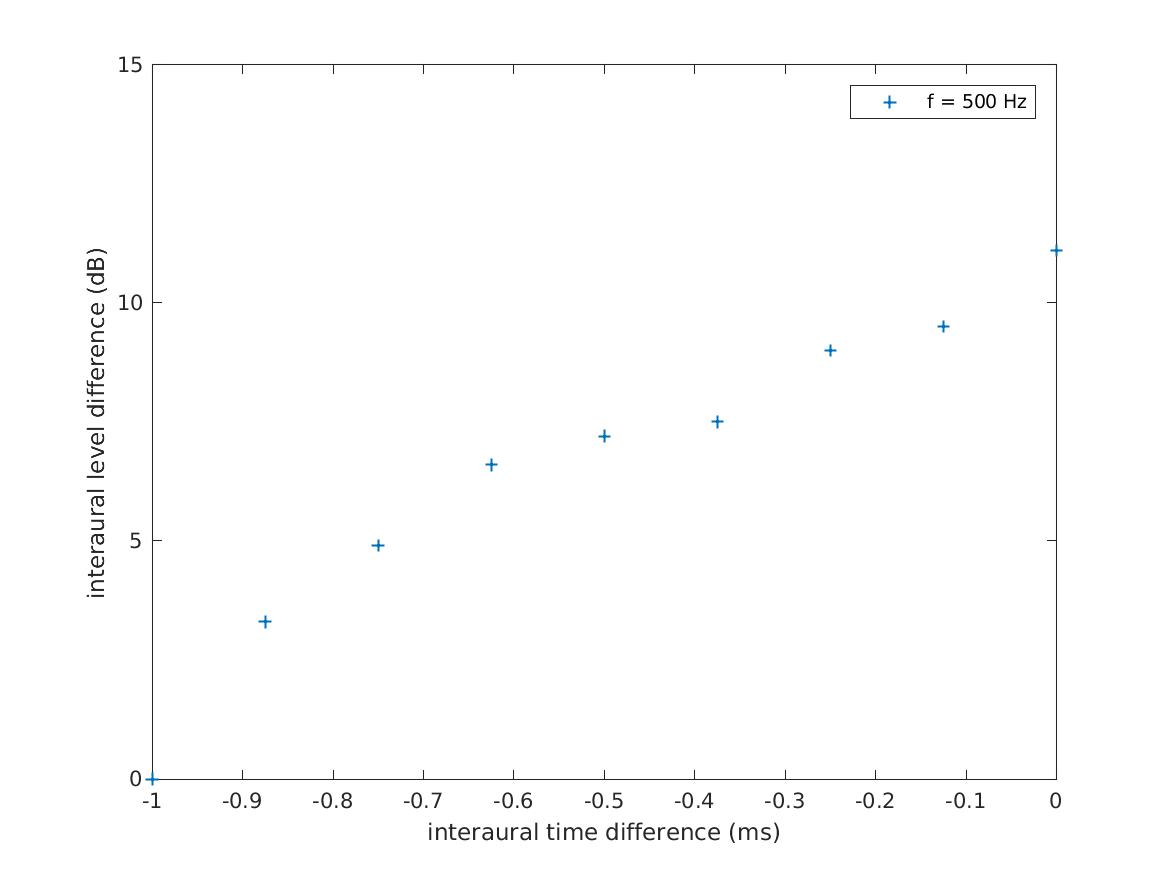Figure 17 can be displayed using

data_lindemann1986('fig17','plot');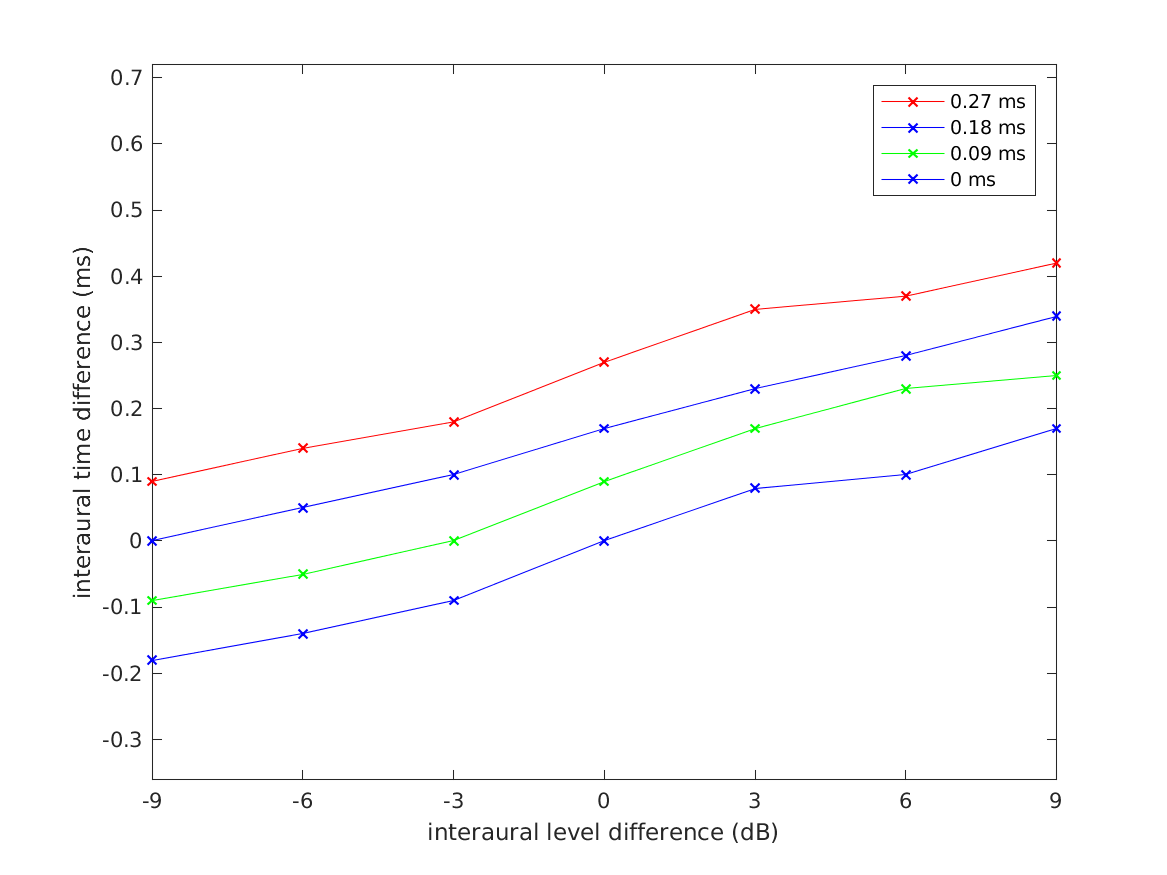## References:

W. Lindemann. Extension of a binaural cross-correlation model by contralateral inhibition. I. Simulation of lateralization for stationary signals. J. Acoust. Soc. Am., 80:1608--1622, 1986.